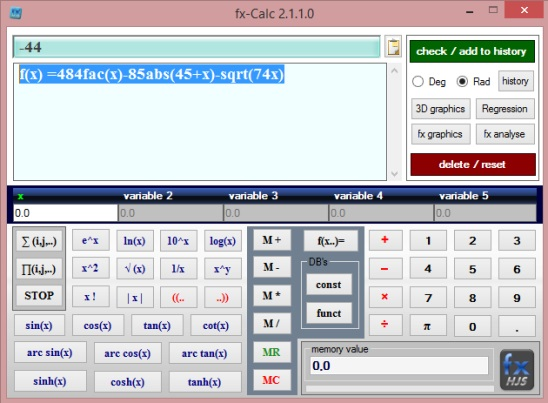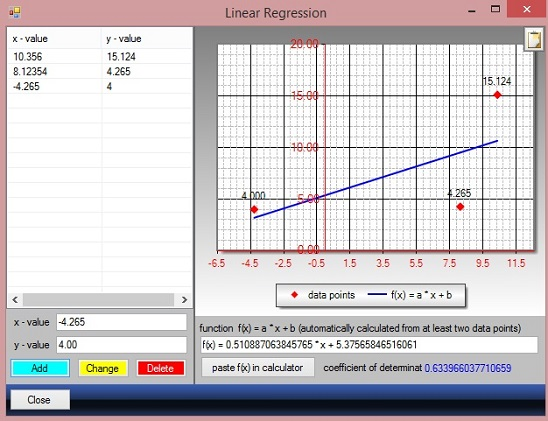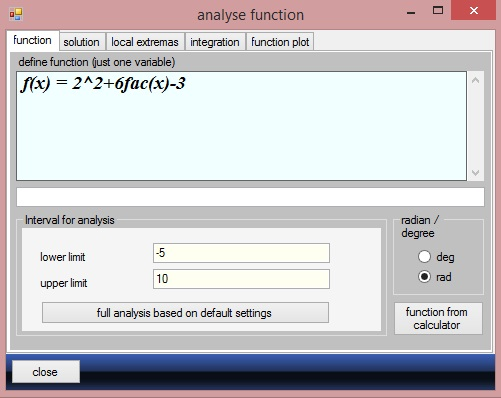Editor Ratings:
User Ratings:
[Total: 0 Average: 0]

fxClac is a free function calculator and function grapher. It is a lightweight and handy function grapher and function calculating freeware, useful for students and mathematician. Using it you can solve complex function equations with just a single click. This freeware lets you solve functions with up to five variables. You can use it to solve almost any math function equation containing Euler function, log, square, square root, inverse, factorial, absolute, trigonometry, and exponential etc.

The freeware is not just a function calculator but it is also a function grapher. You can use the freeware to plot a 3D and 2D graphs for function equations. You can use this function grapher to plot Linear Regressions, 3 Dimensional function plot, and One dimensional function plot. Finally, it also lets you analyze functions, which includes finding the result of function, extremas, result for integration of f(x) and |f(x)|, and plotting the graph of given function.## Calculate complex scientific functions using this function calculator and function grapher:

You can also easily plot the graphs for various functions with one and two variables. The main window of the freeware, looks very much like a scientific calculator. All the numerical, trigonometric, log, factorial, and other buttons are right on the interface. You can use the main window to basically calculate complex function equations. Here, you can calculate a function containing up to five variables. Simply enter the desired function equation in the function input box. You can only solve one equation at a time.

Good thing is that you can either simply enter the equation with keeping the variables as unknown (that is in x,y,z etc.) or you can also enter the values for variables, using the variables fields (just below equation filed on interface). Also the freeware automatically changes the equations color to red from black, if the equation is incomplete or wrong. Simply enter the equation and the freeware gives real-time result, as you type.

The freeware is also a function grapher. There are buttons on main window to plot 3 types of graphs and one button to do complete analysis of a function. These buttons are: 3D graphics, regression, fx graphics, and fx analyse.The 3D graphics opens a sub-window, that lets you plot a 3D graph functions having two variables. Simply enter the equation and then give the upper and lower limits value and, click on Visualize. You’ll get a 3D graph auto-plotted. You can rotate the 3D graphs and move it as well.Regression button opens a new sub-window to solve a liner regression function having two variables: x and y, with both having multiple values. Simply enter the values by typing in x and y value fields and using Add, Change, and Delete button. The graph gets auto-plotted as you enter the values, and a function equation for entered values also gets auto-generated.Clicking on fx graphics button in main window, opens a sub-window to calculate and plot multiple functions at a time. But each function equation should have only one ‘x’ variable. Simply enter the equations one by one and click on Add to plot area button. When done adding functions, click on Plot area tab, and all the equations gets plotted in a graph with different colors.Finally clicking on fx analyse button opens a sub window to calculate and analyze a single function equation containing one variable. Simply enter the equation, next click on Solution tab in same window and click on Run scan button. To get extremas of entered equation click on Local Extremas tab and click on Run scan again. Same is the process of calculating integration values and plot the function.## Key features of this function calculator:

• Completely free.
• Good function calculator and function grapher.
• Quick and easy.
• Lightweight.

Here are some more good math based freeware, you should check: Xfunc, CompliCalc, and Mathcad Express.

## Conclusion:

fxCalc is a good freeware to plot function graphs and solve function equations. It does helps you in solving complex function equations.